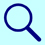PhysiologyWeb  Home  |  FAQ  |  About  |  Contact  |   - Sign InGlossary of Physiology TermsThere are 5 glossary search results for:   Equilibrium potential

Abbreviation:
Veq. or Eeq.

Definition:
Refers to the membrane potential at which there is no net movement of an ion across the plasma membrane into or out of the cell.

Resting Membrane Potential - Establishment of the Membrane Potential
Resting Membrane Potential - Nernst Equilibrium Potential
Nernst Potential Calculator

Abbreviation:
VDF

Definition:
When an ion is not at its electrochemical equilibrium, an electrochemical driving force (VDF) acts on the ion, causing the net movement of the ion across the membrane down its own electrochemical gradient.

The electrochemical driving force is generally expressed in millivolts and is calculated according the following equation:

VDF = VmVeq

where VDF is the electrochemical driving force, Vm is the membrane potential, and Veq is the equilibrium potential.

Related glossary terms/phrases:
Membrane potential
Equilibrium potential

Resting Membrane Potential - Electrochemical Driving Force Acting on Ions
Electrochemical Driving Force Calculator

Definition:
Refers to the balance of chemical and electrical gradients that act on an ion, particularly as it relates to the movement of an ion across a biological membrane (see Resting Membrane Potential - Establishment of the Membrane Potential and Resting Membrane Potential - Nernst Equilibrium Potential).

Related glossary terms/phrases:

Definition:
The Hodgkin cycle represents a positive feedback loop in neurons, where an initial membrane depolarization from the resting value (∼ −70 mV) to the threshold value (∼ −50 mV) leads to rapid depolarization of the membrane potential to approach the equilibrium potential for Na+ (VNa ≈ +60 mV). The voltage-gated Na+ channels of neurons are responsible for the Hodgkin cycle.

See the figure depicting the Hodgkin cycle.

Important Features of the Neuronal Action Potential

Definition:
An equation used to calculate the equilibrium potential (Veq.) of an ion. The equilibrium potential for an ion is also referred to as the Nernst potential for that ion. It is the membrane potential at which no net movement of the ion in question occurs across the membrane.where Veq. is the equilibrium potential, R is the universal gas constant, T is the temperature in Kelvin, z is the valence of the ionic species, F is the Faraday's constant, and [X]o and [X]i are the extracellular and intracellular, respectively, concentrations of the ion in question.